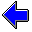Java Security Settings:
This web page employs Java, which requires specific security settings for correct operation.
If the applets on this page do not run correctly, consult the Virtual Chemistry Experiments FAQ
or the Physlet Physics web site for establishing the correct security settings.

# Calorimetry

## Heat Capacity

### Concepts

When heat is transferred to an object, the temperature of the object increases. When heat is removed from an object, the temperature of the object decreases. The relationship between the heat ( q ) that is transferred and the change in temperature ( ΔT ) is

q = C ΔT = C ( Tf - Ti )

The proportionality constant in this equation is called the heat capacity ( C ). The heat capacity is the amount of heat required to raise the temperature of an object or substance one degree. The temperature change is the difference between the final temperature ( Tf ) and the initial temperature ( Ti ).

Quantity Symbol Unit Meaning
heat q joule (J) Energy transfer that produces or results from a difference in temperature
temperature T oC or K Measure of the kinetic energy of molecular motion
temperature change ΔT oC or K Difference between the final and initial temperatures for a process
heat capacity C J oC-1 or J K-1 Heat required to change the temperature of a substance one degree

A calorimeter is an experimental device in which a chemical reaction or physical process takes place. The calorimeter is well-insulated so that, ideally, no heat enters or leaves the calorimeter from the surroundings. For this reason, any heat liberated by the reaction or process being studied must be picked up by the calorimeter and other substances in the calorimeter.

A thermometer is typically inserted in the calorimeter to measure the change in temperature that results from the reaction or physical process. A stirrer is employed to keep the contents of the calorimeter well-mixed and to ensure uniform heating.

The calorimeter shown below contains some water and is equipped with a thermometer, a stirrer, and a heating element. When activated, an electric current is passed through the heating element to generate heat, which is transferred to the calorimeter. In this simulation, you can set the heating rate (units of J sec-1) and the heating time (units of sec).

### Experiment

Objective:

• Determine the heat capacity of the entire calorimeter system (calorimeter and water).

Approach:

• Use the heating element to transfer a known amount of heat to the calorimeter system.
• Observe the temperature of the system before and after the heating process.
• Calculate the change in temperature for the system.
• Calculate the heat capacity of the entire calorimeter system.

### Part 1

In this part of the experiment, the heating element is set to operate for 5 seconds, during which time the heating element will transfer a total of 100 kJ of heat to the calorimeter. To begin the experiment, record the initial temperature, and select the "Start" button to begin the heating process. When the heating process is finished, record the final temperature and calculate the heat capacity of the system.

After running a simulation, it is necessary to reset the system before running another simulation.

### Part 2

Repeat the procedure in Part 1 for determining the heat capacity of the entire calorimeter system, but in this case vary the heat output by the heating element. The heating time is the time the heating element is turned on. The heating rate is the amount of heat the heating element releases per second. Try several different heating times and heating rates. You should obtain the same heat capacity as you did in Part 1.

New values for the heating time and heating rate are processed when the simulation is reset. If you change either value, you must reset the simulation again for the new values to take effect.

 Heating Time sec Heating Rate J sec-1Calorimetry Home PageVirtual Chemistry Home Page

HeatCapacity.html version 2.1
© 2000-2014 David N. Blauch